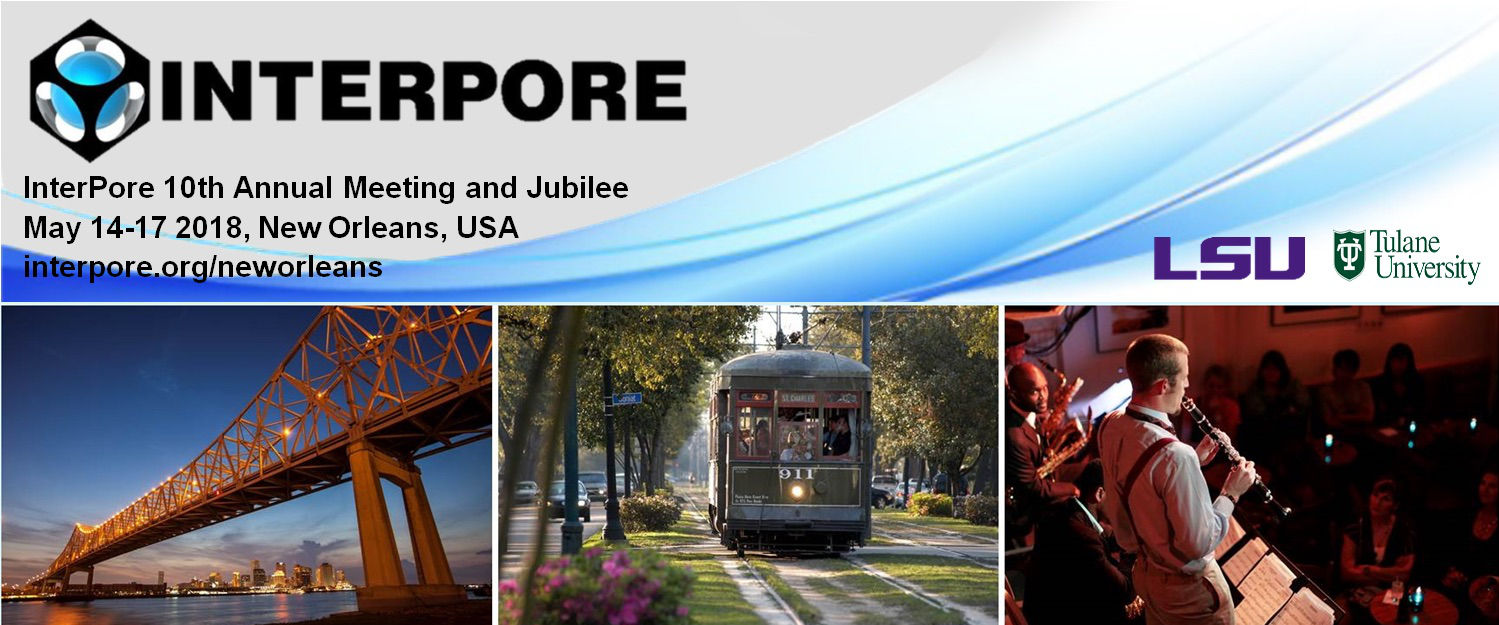#InterPore2018 New Orleans

14-17 May 2018
New Orleans
US/Central timezone

## Propagation of fronts for Richards equation with gravity for general non-equilibrium capillarity pressure conditions

17 May 2018, 08:50
15m
New Orleans

#### New Orleans

Oral 20 Minutes GS 4: Porous media applications (renamed)

### Speaker

Koondanibha Mitra (Eindhoven University of Technology)

### Description

The Richards equation is a commonly used model for unsaturated flow through porous media. Using the Darcy law in the mass balance equation, and bringing the resulting equation to a dimensionless form, for gravity driven flow one gets the equation:
\begin{equation}
\partial_t S(p)= \nabla \cdot \left[ k(S) \left( N_c \nabla p - 1\right)\right].
\end{equation}
Here $N_c$ is the capillary number and $k(S)$ is a nonlinear function that is determined based on experiments. Two unknowns are involved: $S$, the water saturation and $p$, the water pressure. Standard models assume that these are related by a nonlinear relationship determined, again, based on experiments:
\begin{equation}
-p=P_c(S).
\end{equation}
However, models based on this assumption cannot explain phenomena like saturation overshoot or finger formation, which are observed experimentally but ruled out as solutions to the standard Richards equation [DiCarlo (2004), Hassanizadeh & Gray (1993)]. Also, history dependent effects are disregarded [Morrow & Harris (1964)].
In this work we consider non-standard models, where dynamic and hysteretic effects are included in the $p$-$S$ relationship:
\begin{equation}
-p=P^+(S)-P^-(S)\mathrm{sign}(\partial_t S)-\tau f(S)\partial_t S.
\end{equation}
To understand the behavior of this system in the one-dimensional case, we consider travelling wave (TW) solutions [van Duijn et al. (2017)]. We obtain the existence of TW and discuss their qualitative behavior. At first, the effects of capillary hysteresis and dynamic capillarity are considered separately. Then their combined effects on the general behavior of the system is delineated. These resulting solutions exhibit non monotone profiles and saturation overshoots, which is in good agreement with the experiments [DiCarlo (2004)].
Conditions for having saturation overshoots and reaching full saturation are derived and an extension to the existing model is proposed that includes the effect of non-vertical scanning curves. Finally, we present a numerical scheme to solve this mathematical model, discuss its convergence, and present some numerical results validating the theoretical findings.

### References

van Duijn, C. J., Mitra, K., \& Pop, I. S. (2018). Travelling wave solutions for the Richards equation incorporating non-equilibrium effects in the capillarity pressure. Nonlinear Analysis: Real World Applications, 41, 232-268

Hassanizadeh, S. M. and Gray, W. G., Thermodynamic basis of capillary pressure in porous media, Water Resour. Res. 29 (1993): 3389-3405

Morrow, N. R. and Harris, C. C., Capillary equilibrium in porous materials, Soc. Pet. Eng. Jour. (1965)

DiCarlo, D. A. , Experimental measurements of saturation overshoot on infiltration. Water Resour. Res. 40 (2004)